Next: Wave Drag on Ships Up: Waves in Incompressible Fluids Previous: Gravity Waves in Shallow

# Energy of Gravity Waves

It is easily demonstrated, from the analysis contained in the previous sections, that a gravity wave of arbitrary wavenumber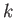, propagating horizontally through water of depth, has a phase velocity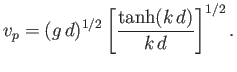(11.43)

Moreover, the ratio of the group to the phase velocity is(11.44)

It follows that neither the phase velocity nor the group velocity of a gravity wave can ever exceed the critical value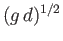. It is also easily demonstrated that the displacement and velocity fields associated with a plane gravity wave of wavenumber, angular frequency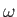, and surface amplitude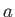, are(11.45)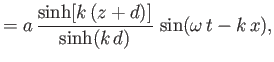(11.46)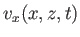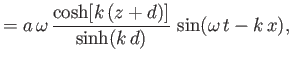(11.47)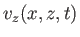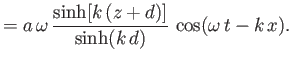(11.48)

The mean kinetic energy per unit surface area associated with a gravity wave is defined(11.49)

where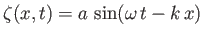(11.50)

is the vertical displacement at the surface, and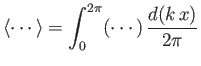(11.51)

is an average over a wavelength. Given that, it follows from Equations (11.47) and (11.48) that, to second order in,(11.52)

Making use of the general dispersion relation (11.21), we obtain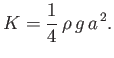(11.53)

The mean potential energy perturbation per unit surface area associated with a gravity wave is defined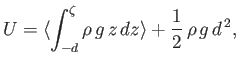(11.54)

which yields(11.55)

or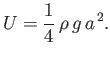(11.56)

In other words, the mean potential energy per unit surface area of a gravity wave is equal to its mean kinetic energy per unit surface area.

Finally, the mean total energy per unit surface area associated with a gravity wave is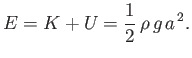(11.57)

This energy depends on the wave amplitude at the surface, but is independent of the wavelength, or the water depth.Next: Wave Drag on Ships Up: Waves in Incompressible Fluids Previous: Gravity Waves in Shallow
Richard Fitzpatrick 2016-03-31